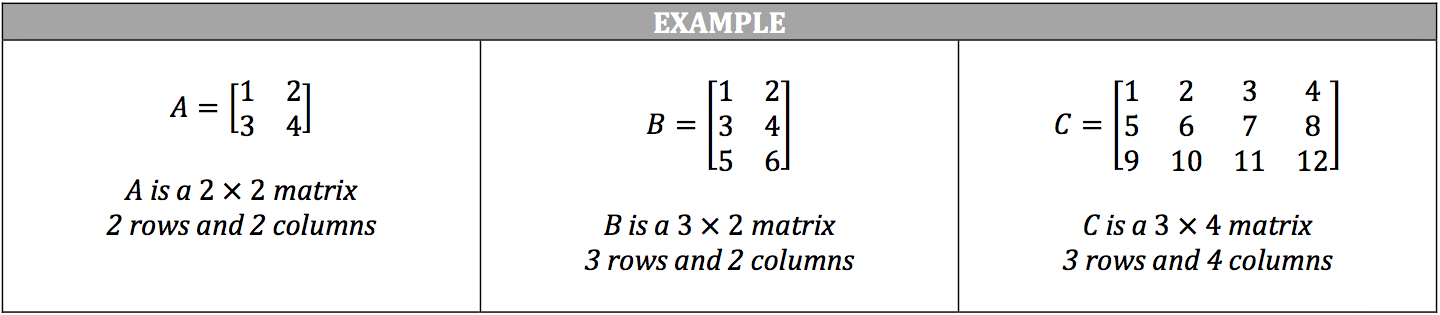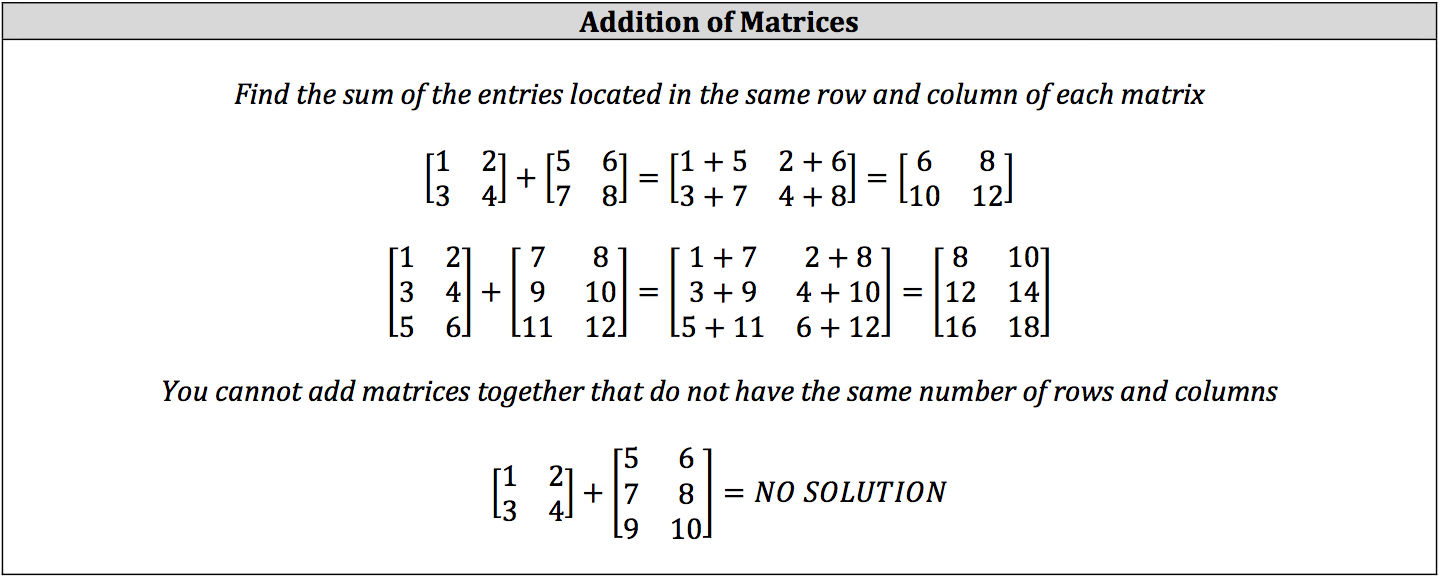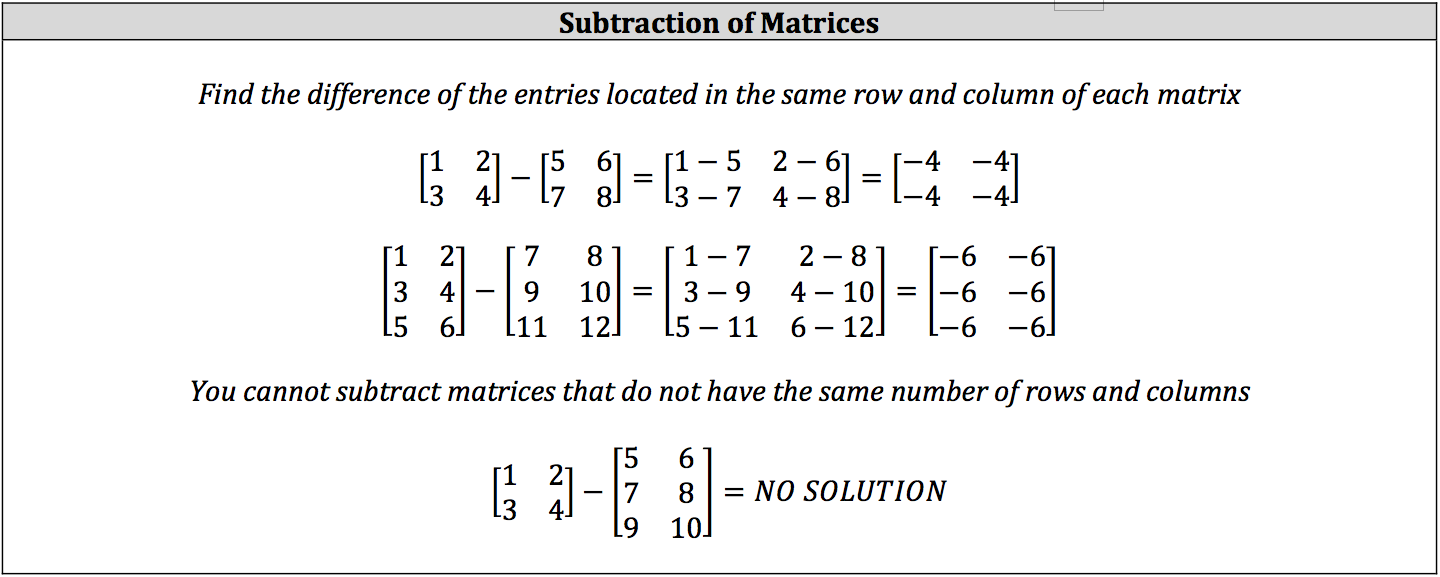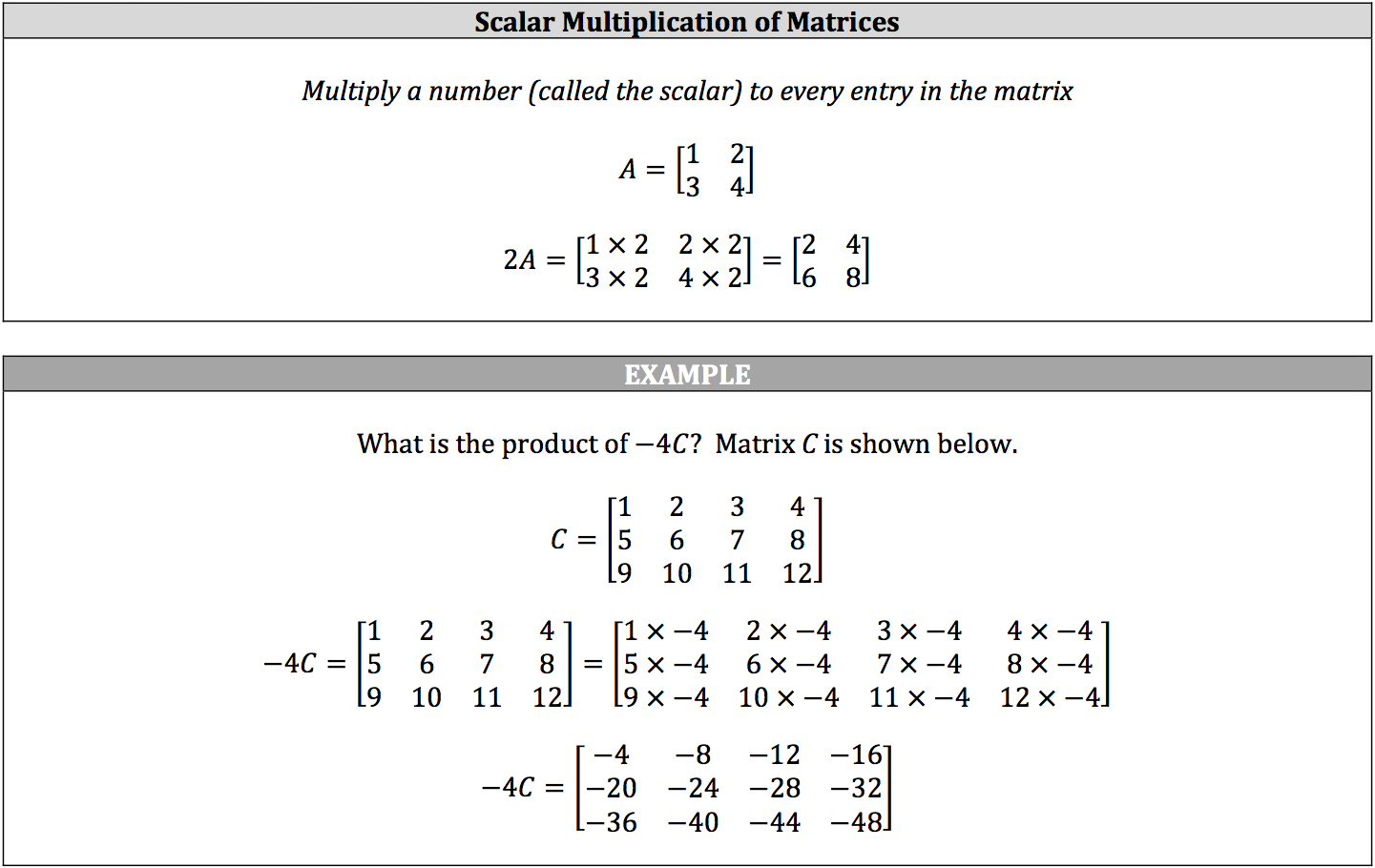A collection of numbers (called entries) contained within a pair of [ ] brackets is called a matrix. A matrix is labeled by a variable, which is usually a capitalized letter. In a sense, matrices are like sets of data, except that a matrix is made up of rows and columns of data as opposed to just one row of data.

A matrix is defined by the numbers of rows it contains by the number of columns it contains (in that order). There are a large number of types and uses for matrices, but you will only need to know how to perform:

• subtraction
• scalar multiplication of matrices